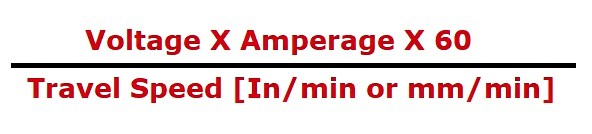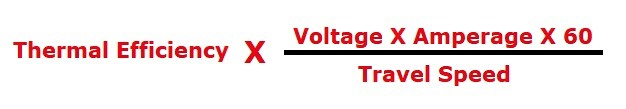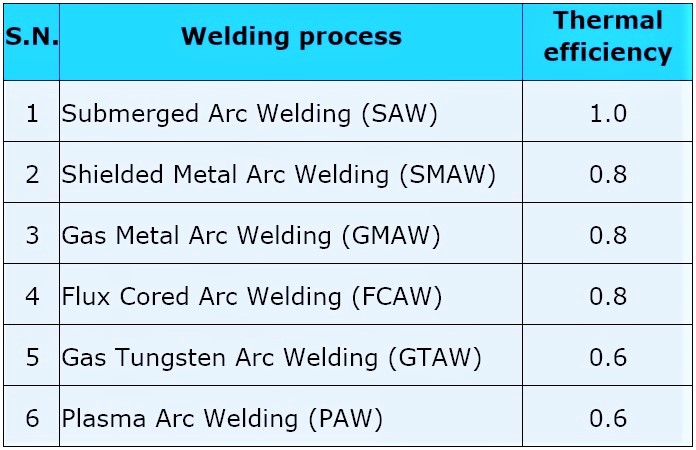# How to calculate heat input

Heat input is a critical parameter for arc welding processes and it must be controlled to ensure sound weld quality.

Heat input may be defined as “The amount of electrical energy that is supplied to a weld during the welding process”.

### How to calculate Heat input?

There are several ways of calculating the heat input. We shall discuss the two most common methods of calculating the heat input.

###(adsbygoogle = window.adsbygoogle || []).push({});

Where,

• Heat input is expressed in J/In (Joule/Inch) or J/mm (Joule/mm)
• Voltage is expressed in Volts
• Travel speed is expressed in In/min or mm/min

The unit for heat input obtained by this formula shall be either in J/In or J/mm. To get the results in KJ/in or KJ/mm, divide the result by 1000.

Example 1: If a welder takes 2 minutes to complete an 18 inches long weld. He keeps the voltage at 24 volts and the current 120 amps. What is your heat input?

Given data parameters are;

Travel Speed = Length of Weld/Time to weld = 18 inches/2 minutes = 9 in/min

Voltage = 24 volts

Current = 120 amps

Heat Input = (24 X 120 X 60)/ 9 = 19200 J/in

= 19.2 KJ/in (Divided by 1000 to obtain the result in KJ/in.)

##### 2. European system (given in EN ISO 1011-1 and PD ISO/TR 18491)

An additional parameter of Thermal efficiency (process efficiency or arc efficiency) is used while calculating heat input as per European standards.

###Value of thermal efficiency is different for different arc welding processes, see table 1:

Table – 1### Why Heat input is so important?

Heat input affects the cooling rates in welds and thereby it affects the microstructure of the weld metal and that of the heat-affected zone (HAZ). A change in microstructure directly affects the mechanical properties of weld metal and heat-affected zone (HAZ). Therefore, it’s very important to control the heat input to achieve a sound microstructure and a good quality weld.

Note: The heat input (as calculated by ASME standards) is referred to as “arc energy” under the European system. Hence the following formula holds true with regard to the European system;

Heat input = Thermal efficiency X Arc energy

### 15 thoughts on “How to calculate heat input”

1.Hai sir please explain about post weld heat treatment PWHT for pressure vessels.

2.3.Dear sir please post about line checking in Piping ,mechanical punching and punch closing procedures…

4.Dear sir,
Today I visited your website. I got very usefull notes. Thanks for your usefull notes.
Regards,
Dharam Tyagi

5.Related to pipings

6.Very nice information regarding heat input. Can you please tell the maximum and minimum values of heat input for different grades of materials based on ASME Standards.

7.8.The best part of your article is , ” Simple” and To the point which makes it easy to understand.

9.MAHESH KUMAR PA

Very Nice publication

10.I have been testing, this is one area I have had issues with. After this post, I get it!!! And you even cleared up K/in and KJ/in… Thank you very very much!!!

11.Dear sir,
Today I visited your website. I got very useful Information on your website . Thanks for your useful information.
Regards,

12.When thickness of the weld is given, how do we calculate for heat input

13.Please also tell the technique to select the welding electrode.

14.Heat input maximum and minimum in ASME how much any welding prices wher to find

15.How to calculate heat input in KJ/mm, when current and voltage given in range, like current 79-81 amp & voltage is given 27-29 volts and travel speed is equal to 135 mm/min.